Get 1 free lesson per week // Add a new lessonNew account
Millions of accounts created on our sites.

• Home
• Print
• Guestbook
• Report a bug

•Get a free English lesson every week!

Partners:
- Our other sites

Learn English > English lessons and exercises > English test #128904: Vocabulary: arithmetic and counting> Other English exercises on the same topic: Numbers [Change theme] > Similar tests: - Cardinal and ordinal numbers - Fractions and percentages - ESL-Numbers from 1 to 10 - Geometric shapes - Vocabulary: mathematics - Geometry-Vocabulary - Idioms: numbers - Numbers and dates... (Level 1) > Double-click on words you don't understand

# Vocabulary: arithmetic and counting

The only sound of the words 'mathematics' or 'arithmetic' gives some of us the shivers... Yet, some

of these notions are useful in our daily lives, and we can't do without basic knowledge of them.

The optimists and the people who love maths say that it's a 'universal language'! Therefore if you

attend a math lesson in a foreign country, you're most likely to understand and be able to participate!

This is good news, isn't it?Statistics is important...

 Mathematics + singular maths (GB)/ math (US) mathematical/ a mathematician statistics (singular) statistics (plural) statistical/ a statistician arithmetic(al)/ algebraic arithmetic/ counting a sign/ a symbolA number An even/ odd number A whole number/ an integer A prime number A figure/ a numeral A digit/ a figure A four-figure number A four-digit number A decimal The decimal point A calculation A sum Mental arithmetic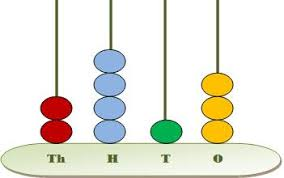2, 413

- To write out a number in figures

- To calculate/ to reckon/ to compute/ to work out

- To count up to ten

 A factor a variable A formula a constant An equation a function A unit a logarithm A problem To solve The answer/ the solution To count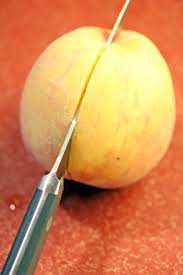To halve an apple

- What do you reckon?

- by my reckoning/ according to my calculations

- I get 406/ I make it 406

- your calculations are out/ are wrong

 Addition A substraction An addition/ a sum To take away from To add up/ to reckon up To substract from plus/ a plus minus/ a minus sign Multiplication Division To multiply by To divide 12 by 6/ 6 into 12 Multiplication tables indivisible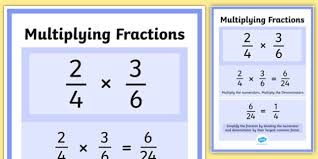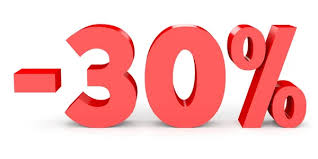- 12 plus 8 is 20// 12 and 8 make 20

- 20 minus 2 is 12

- The three/ four times table

- It won't divide by 5/ It's not divisible by 5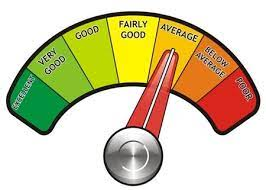To equal/ to be equal to To amount to / to total To carry Double/ to double treble/ to treble A percentage per cent a ratio proportional to the average/ on average the square root A common fraction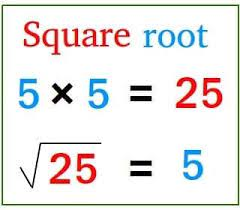- One half/ one third/ two thirds

- four and a half

I hope I didn't give you a headache...I hope this lesson will be useful to you one day!

Go for the test!Intermediate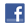Twitter Share
English exercise "Vocabulary: arithmetic and counting" created by here4u with The test builder. [More lessons & exercises from here4u]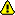Please log in to save your progress.

1. 'In your English exercise, you are given sentences with numbers written in full letters. Youll have to . Easy, isnt it?'

2. It took the student half an hour to this difficult problem

3. 'As youve helped me, the fair solution is to our gain: you agree, dont you?'

4. 'My new bank code is : I have chosen your date of birth'

5. 'Your exercise is wrong! You have found 6.48 and it says the answer is an '

6. 'Ive always been good at and loved it when we did that at school.'

7. 'Would you rather have of this delicious chocolate cake or half of it?' 'I give you two guesses'

8. 'No, the result cant be 542! I said the result was an !'

9. 'You've miscalculated... Look! ... that's where your mistake is! '

10. This exercise was a success! The mark of the students of this group was exceptionally high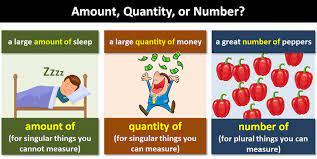End of the free exercise to learn English: Vocabulary: arithmetic and counting
A free English exercise to learn English.
Other English exercises on the same topic : Numbers | All our lessons and exercises